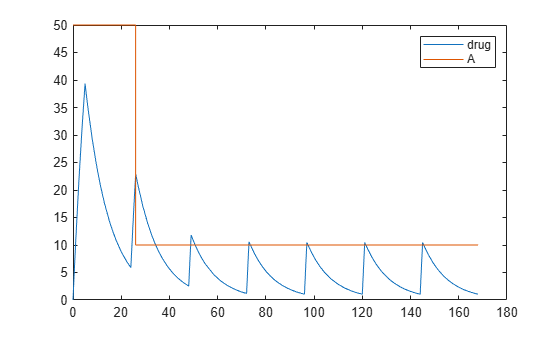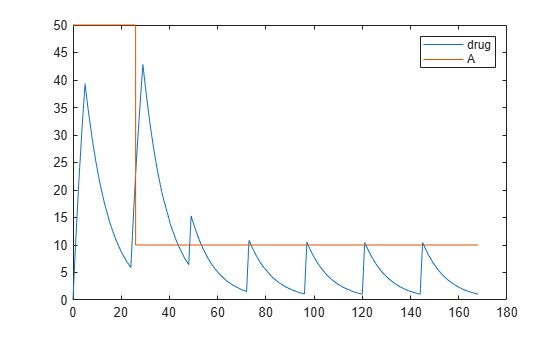# RepeatDose object

Define drug dosing protocol

## Description

A `RepeatDose `object defines a series of doses to the amount of a species during a simulation. The `TargetName` property of a dose object defines the species that receives the dose.

Each dose is the same amount, as defined by the `Amount` property, and given at equally spaced times, as defined by the `Interval` property. The `RepeatCount` property defines the number of injections in the series, excluding the initial injection. The `Rate` property defines how fast each dose is given.

To use a dose object in a simulation you must add the dose object to a model object and set the `Active` property of the dose object to true. Set the `Active` property to true if you always want the dose to be applied before simulating the model.

### Warning

The `Active` property of the `RepeatDose object` will be removed in a future release. Explicitly specify a dose or an array of doses as an input argument when you simulate a model using `sbiosimulate`.

When there are multiple active RepeatDose objects on a model and if there are duplicate specifications for a property value, the last occurrence for the property value in the array of dose, is used during simulation. You can find out which dose is applied last by looking at the indices of the dose objects stored on the model.

You can set these dose properties to model parameters: `Amount`, `Interval`, `Rate`, `RepeatCount`, `StartTime`, `LagParameterName` and `DurationParameterName`. You can set these properties, except `LagParameterName` and `DurationParameterName`, to either a numeric value or the name of a model-scoped parameter (as a character vector or string). Parameterizing dose properties provides more flexibility for different dosing applications, such as scaling the dose amount by body weight. For details, see Parameterized and Adaptive Doses.

## Constructor Summary

 sbiodose Construct dose object

## Method Summary

Methods for `RepeatDose` objects

 copyobj Copy SimBiology object and its children delete Delete SimBiology object display Display summary of SimBiology object get Get SimBiology object properties getTable(ScheduleDose,RepeatDose) Return data from SimBiology dose object as table rename Rename object and update expressions set Set SimBiology object properties setTable(ScheduleDose,RepeatDose) Set dosing information from table to dose object

## Property Summary

Properties for `RepeatDose` objects

 Active Indicate object in use during simulation Amount Amount of dose AmountUnits Dose amount units DurationParameterName Parameter specifying length of time to administer a dose EventMode Determine how events that change dose parameters affect in-progress dosing Interval Time between doses LagParameterName Parameter specifying time lag for dose Name Specify name of object Notes HTML text describing SimBiology object Parent Indicate parent object Rate Rate of dose RateUnits Units for dose rate RepeatCount Dose repetitions StartTime Start time for initial dose time Tag Specify label for SimBiology object TargetName Species receiving dose TimeUnits Show time units for dosing and simulation Type Display SimBiology object type UserData Specify data to associate with object

## Examples

collapse all

Parameterize the `Amount` property of a dose to scale it by the body weight of a patient.

Create a simple model with linear elimination and an amount parameter.

```model = sbiomodel('simple model'); compartment = addcompartment(model,'Central',1); compartment.CapacityUnits = 'liter'; species = addspecies(model,'drug'); species.InitialAmountUnits = 'milligram'; % Elimination rate elimParam = addparameter(model,'kel',0.1); elimParam.ValueUnits = '1/hour'; % Elimination reaction reaction = addreaction(model,'drug -> null'); reaction.ReactionRate = 'kel*drug'; amountParam = addparameter(model,'A',50); amountParam.ConstantValue = false; amountParam.ValueUnits = 'milligram'```
```amountParam = SimBiology Parameter Array Index: Name: Value: Units: 1 A 50 milligram ```

Create a dose with its `Amount` property set to the amount parameter '`A'`.

```dose = adddose(model,'adaptive dose','repeat'); dose.Amount = 'A';```

Set other dose properties.

```dose.TargetName = 'drug'; dose.StartTime = 0; dose.TimeUnits = 'hour'; dose.Interval = 24; dose.RepeatCount = 7;```

Add a parameter to represent the body weight.

```weightParam = addparameter(model,'weight', 80); weightParam.ValueUnits = 'kilogram';```

Scale the dose amount by the body weight using an initial assignment rule.

```scaleParam = addparameter(model,'doseAmountPerWeight',0.6); scaleParam.ValueUnits = 'milligram/kilogram'; rule = addrule(model,'A = weight*doseAmountPerWeight','initialAssignment');```

Simulate the model for 7 days and plot the results.

```configset = getconfigset(model); configset.StopTime = 7*24; configset.TimeUnits = 'hour'; [time, drugAndAmount] = sbiosimulate(model,dose); plot(time, drugAndAmount); legend('drug','A');```Create a simple model with linear elimination, an amount parameter, and a rate parameter.

```model = sbiomodel('simple model'); compartment = addcompartment(model,'Central',1); compartment.CapacityUnits = 'liter'; species = addspecies(model,'drug'); species.InitialAmountUnits = 'milligram'; % Elimination rate elimParam = addparameter(model,'kel',0.1); elimParam.ValueUnits = '1/hour'; % Elimination reaction reaction = addreaction(model,'drug -> null'); reaction.ReactionRate = 'kel*drug'; % Add amount and rate parameters amountParam = addparameter(model,'A',50); amountParam.ConstantValue = false; amountParam.ValueUnits = 'milligram'```
```amountParam = SimBiology Parameter Array Index: Name: Value: Units: 1 A 50 milligram ```
```rateParam = addparameter(model,'R',10); rateParam.ValueUnits = 'milligram/hour'```
```rateParam = SimBiology Parameter Array Index: Name: Value: Units: 1 R 10 milligram/hour ```

Create a dose with its `Amount` and `Rate` properties set to the amount and rate parameters 'A' and 'R', respectively.

```dose = adddose(model,'adaptive dose','repeat'); dose.Amount = 'A'; dose.Rate = 'R';```

Set other dose properties.

```dose.TargetName = 'drug'; dose.StartTime = 0; dose.TimeUnits = 'hour'; dose.Interval = 24; dose.RepeatCount = 7;```

Prepare the configuration set to simulate the model for 7 days.

```configset = getconfigset(model); configset.StopTime = 7*24; configset.TimeUnits = 'hour';```

Add an event to reset the dose amount to 10 at time >= 26.

`event = addevent(model,'time >= 26','A = 10');`

Set the `EventMode` property to 'stop'. This setting causes any ongoing dose event to stop at 26 hours.

`dose.EventMode = 'stop';`

Simulate the model. The second dose event stops at 26 hours, and the subsequent dose events continue with the new dose amount of 10.

```[time, drugAndAmount] = sbiosimulate(model,dose); figure plot(time, drugAndAmount); legend('drug','A');```Alternatively, you can allow the ongoing dose event to finish before applying the new dose amount by setting `EventMode` to 'continue'.

`dose.EventMode = 'continue';`

Simulate the model. In this case, the second dose event continues to 26 hours.

```[time, drugAndAmount] = sbiosimulate(model,dose); figure plot(time, drugAndAmount); legend('drug','A');```